The function aggregate_profiles() calculates an aggregate of ceteris paribus profiles. It can be: Partial Dependence Profile (average across Ceteris Paribus Profiles), Conditional Dependence Profile (local weighted average across Ceteris Paribus Profiles) or Accumulated Local Dependence Profile (cummulated average local changes in Ceteris Paribus Profiles).

aggregate_profiles(
x,
...,
variable_type = "numerical",
groups = NULL,
type = "partial",
variables = NULL,
span = 0.25,
center = FALSE
)

## Arguments

x a ceteris paribus explainer produced with function ceteris_paribus() other explainers that shall be calculated together a character. If numerical then only numerical variables will be calculated. If categorical then only categorical variables will be calculated. a variable name that will be used for grouping. By default NULL which means that no groups shall be calculated either partial/conditional/accumulated for partial dependence, conditional profiles of accumulated local effects if not NULL then aggregate only for selected variables will be calculated smoothing coeffcient, by default 0.25.It's the sd for gaussian kernel by default accumulated profiles start at 0. if center=TRUE then they are centered around average response

## Value

an object of the class aggregated_profiles_explainer

Explanatory Model Analysis. Explore, Explain and Examine Predictive Models. https://pbiecek.github.io/ema

## Examples

library("DALEX")
library("randomForest")

model_titanic_rf <- randomForest(survived ~ .,  data = titanic_imputed)#> Warning: The response has five or fewer unique values.  Are you sure you want to do regression?
explain_titanic_rf <- explain(model_titanic_rf,
data = titanic_imputed[,-8],
y = titanic_imputed[,8])#> Preparation of a new explainer is initiated
#>   -> model label       :  randomForest  (  default  )
#>   -> data              :  2207  rows  7  cols
#>   -> target variable   :  2207  values
#>   -> predict function  :  yhat.randomForest  will be used (  default  )
#>   -> predicted values  :  numerical, min =  0.01309591 , mean =  0.3218617 , max =  0.9905597
#>   -> model_info        :  package randomForest , ver. 4.6.14 , task regression (  default  )
#>   -> residual function :  difference between y and yhat (  default  )
#>   -> residuals         :  numerical, min =  -0.7721227 , mean =  0.0002950252 , max =  0.9013245
#>   A new explainer has been created!
selected_passangers <- select_sample(titanic_imputed, n = 100)
cp_rf <- ceteris_paribus(explain_titanic_rf, selected_passangers)
#>        gender age            class    embarked    fare sibsp parch    _yhat_
#> 515    female  45              2nd Southampton 10.1000     0     0 0.8502254
#> 515.1    male  45              2nd Southampton 10.1000     0     0 0.1010224
#> 604    female  17              3rd Southampton  7.1701     1     0 0.4409059
#> 604.1    male  17              3rd Southampton  7.1701     1     0 0.1154397
#> 1430   female  25 engineering crew Southampton  0.0000     0     0 0.6807248
#> 1430.1   male  25 engineering crew Southampton  0.0000     0     0 0.2426040
#>        _vname_ _ids_      _label_
#> 515     gender   515 randomForest
#> 515.1   gender   515 randomForest
#> 604     gender   604 randomForest
#> 604.1   gender   604 randomForest
#> 1430    gender  1430 randomForest
#> 1430.1  gender  1430 randomForest
#>
#>
#> Top observations:
#>      gender age            class    embarked    fare sibsp parch    _yhat_
#> 515    male  45              2nd Southampton 10.1000     0     0 0.1010224
#> 604    male  17              3rd Southampton  7.1701     1     0 0.1154397
#> 1430   male  25 engineering crew Southampton  0.0000     0     0 0.2426040
#> 865    male  20              3rd   Cherbourg  7.0406     0     0 0.1168150
#> 452  female  17              3rd  Queenstown  7.1408     0     0 0.6270921
#> 1534   male  38 victualling crew Southampton  0.0000     0     0 0.1550319
#>           _label_ _ids_
#> 515  randomForest     1
#> 604  randomForest     2
#> 1430 randomForest     3
#> 865  randomForest     4
#> 452  randomForest     5
#> 1534 randomForest     6
# continouse variable
pdp_rf_p <- aggregate_profiles(cp_rf, variables = "age", type = "partial")
pdp_rf_p$_label_ <- "RF_partial" pdp_rf_c <- aggregate_profiles(cp_rf, variables = "age", type = "conditional") pdp_rf_c$_label_ <- "RF_conditional"
pdp_rf_a <- aggregate_profiles(cp_rf, variables = "age", type = "accumulated")
pdp_rf_a$_label_ <- "RF_accumulated" plot(pdp_rf_p, pdp_rf_c, pdp_rf_a, color = "_label_")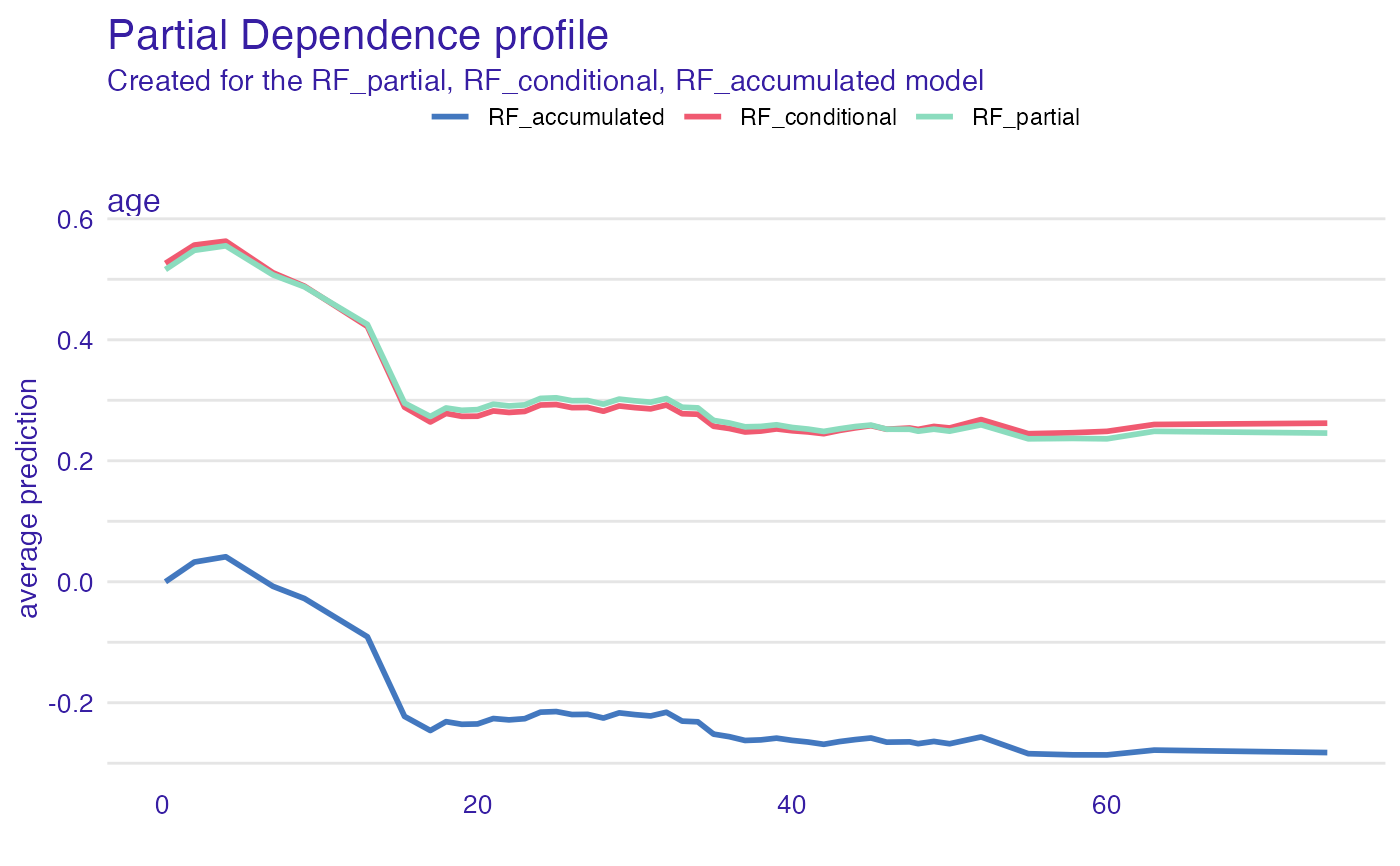# \donttest{ pdp_rf <- aggregate_profiles(cp_rf, variables = "age", groups = "gender") head(pdp_rf)#> Top profiles : #> _vname_ _label_ _x_ _groups_ _yhat_ _ids_ #> 1 age randomForest_female 0.1666667 female 0.7293980 0 #> 2 age randomForest_female 2.0000000 female 0.7184480 0 #> 3 age randomForest_female 4.0000000 female 0.7409075 0 #> 4 age randomForest_female 7.0000000 female 0.7212749 0 #> 5 age randomForest_female 9.0000000 female 0.7276165 0 #> 6 age randomForest_female 13.0000000 female 0.7450006 0plot(cp_rf, variables = "age") + show_observations(cp_rf, variables = "age") + show_rugs(cp_rf, variables = "age", color = "red") + show_aggregated_profiles(pdp_rf, size = 3, color = "_label_")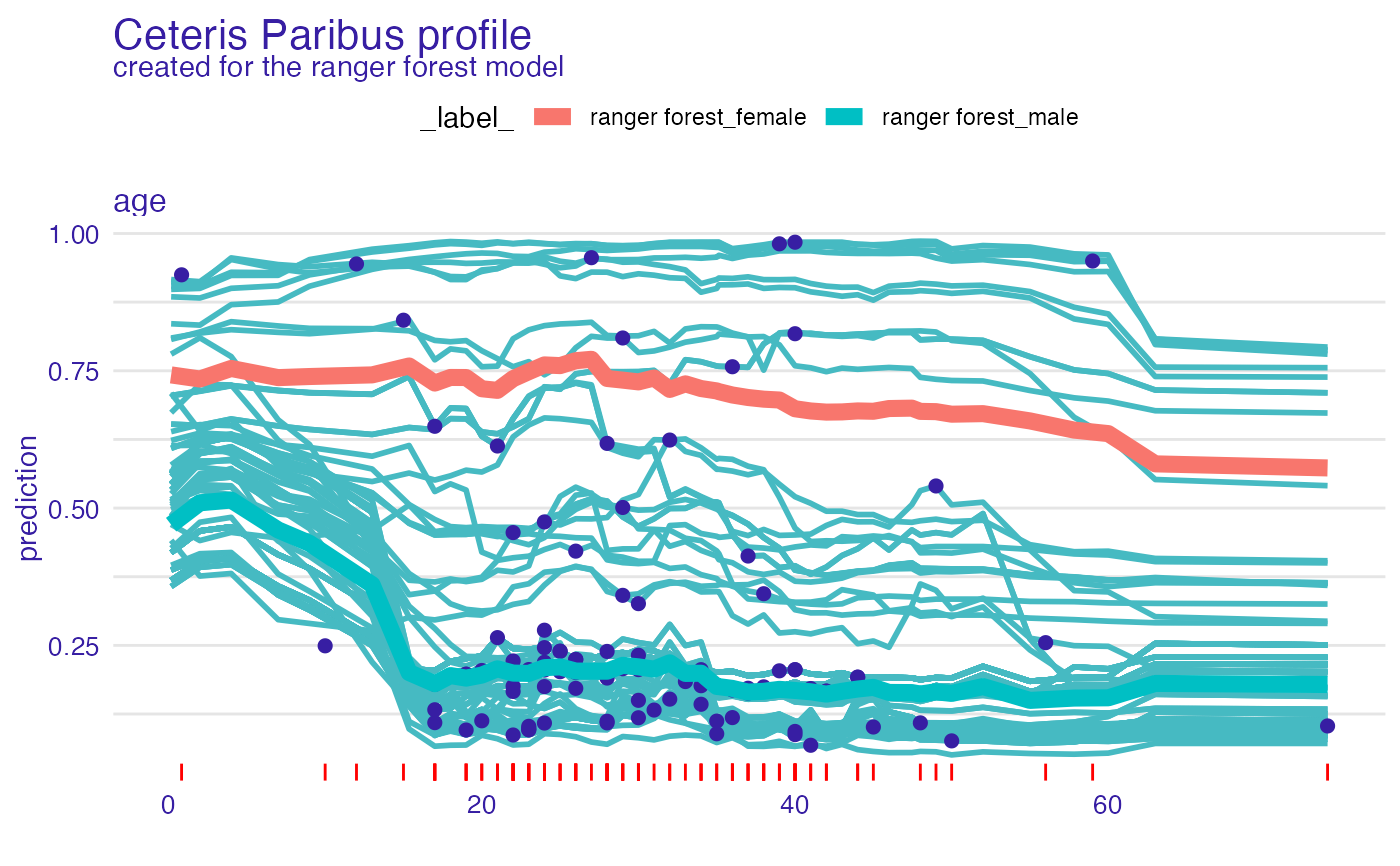# categorical variable pdp_rf_p <- aggregate_profiles(cp_rf, variables = "class", variable_type = "categorical", type = "partial") pdp_rf_p$_label_ <- "RF_partial"
pdp_rf_c <- aggregate_profiles(cp_rf, variables = "class",
variable_type = "categorical", type = "conditional")
pdp_rf_c$_label_ <- "RF_conditional" pdp_rf_a <- aggregate_profiles(cp_rf, variables = "class", variable_type = "categorical", type = "accumulated") pdp_rf_a$_label_ <- "RF_accumulated"
plot(pdp_rf_p, pdp_rf_c, pdp_rf_a, color = "_label_")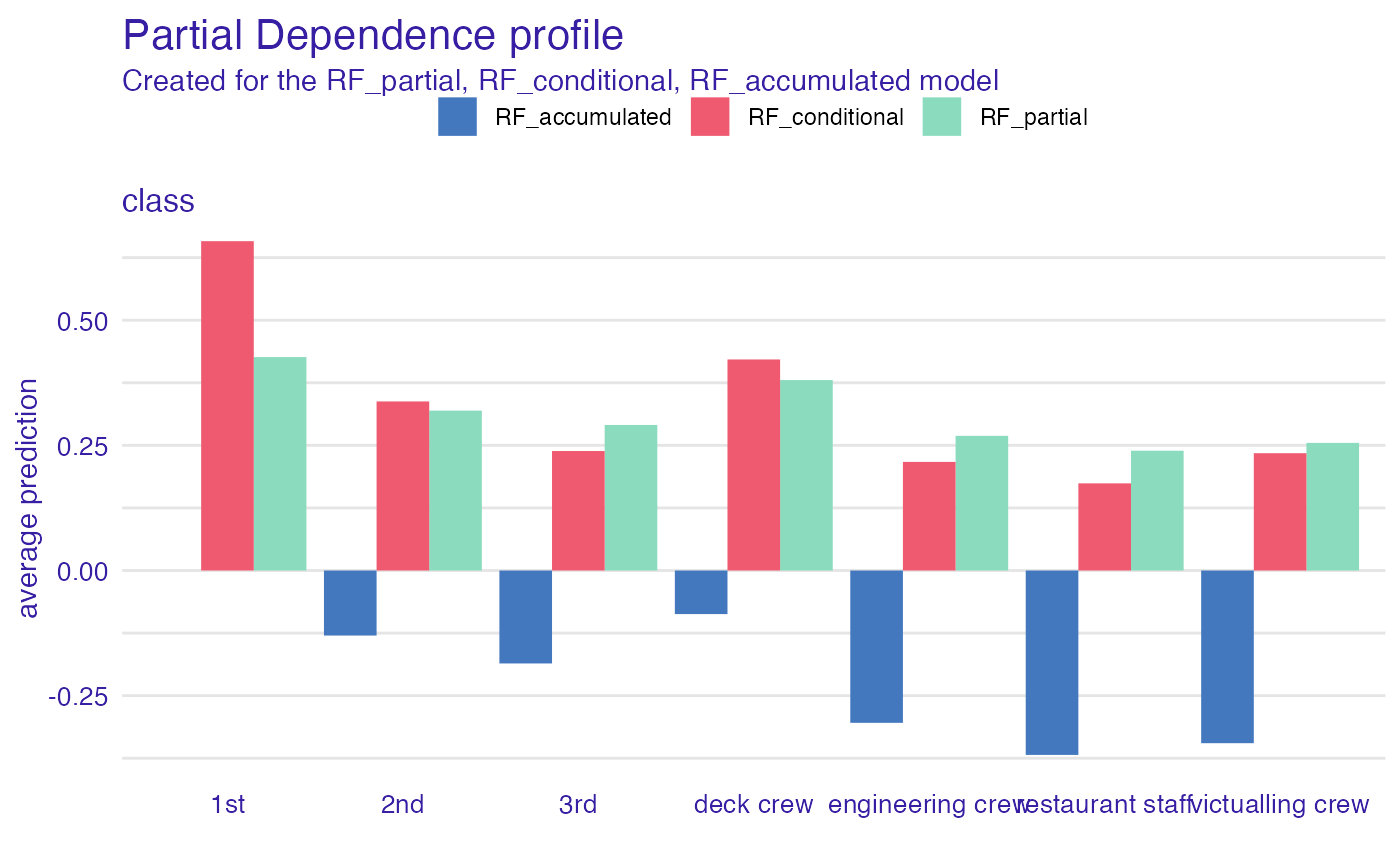# or maybe flipped?
library(ggplot2)#>
#> Attaching package: ‘ggplot2’#> The following object is masked from ‘package:randomForest’:
#>
#>     marginplot(pdp_rf_p, pdp_rf_c, pdp_rf_a, color = "_label_") + coord_flip()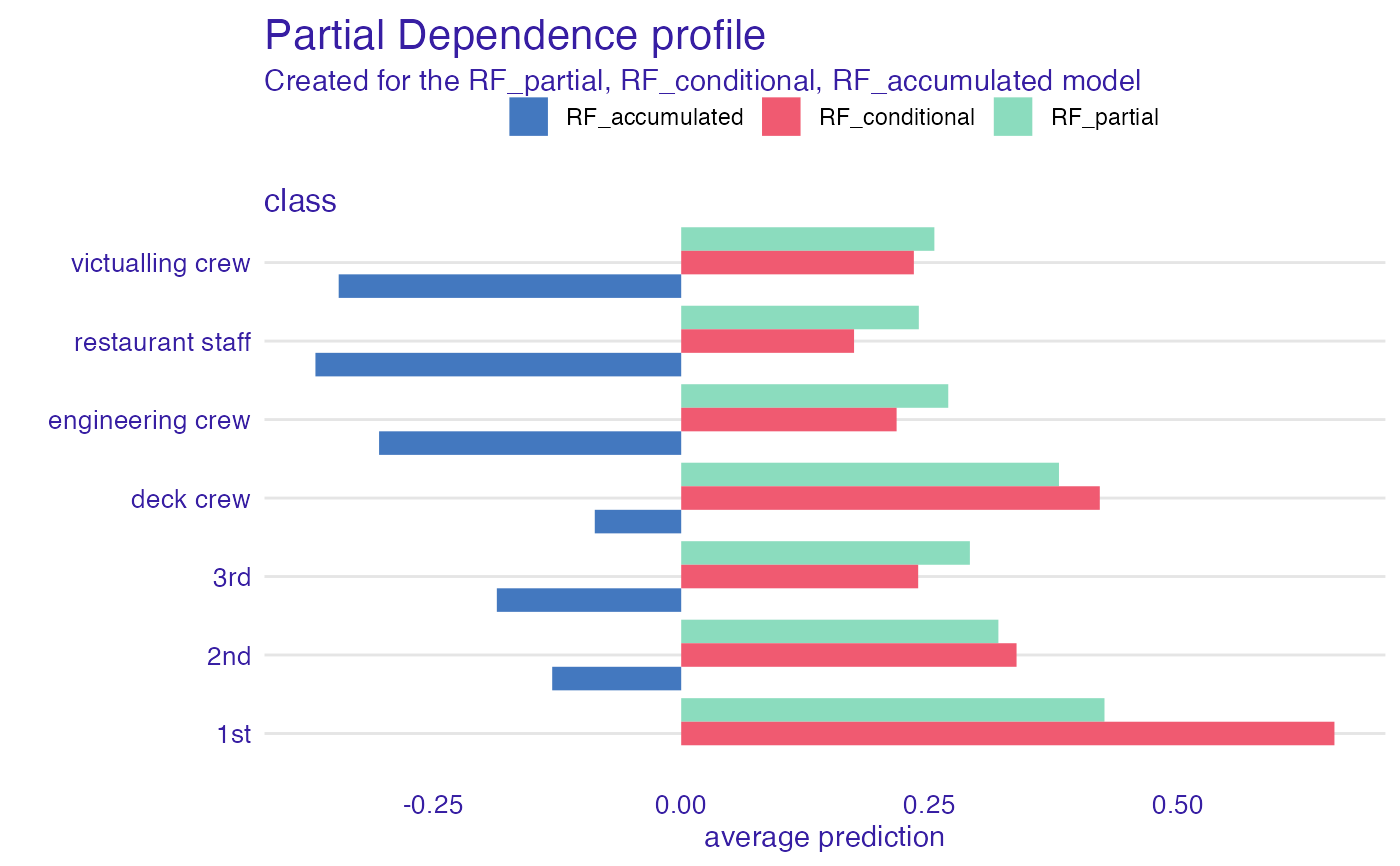pdp_rf <- aggregate_profiles(cp_rf, variables = "class", variable_type = "categorical",
groups = "gender")
#> 10   class   randomForest_male 3rd     male 0.1655832     0plot(pdp_rf, variables = "class")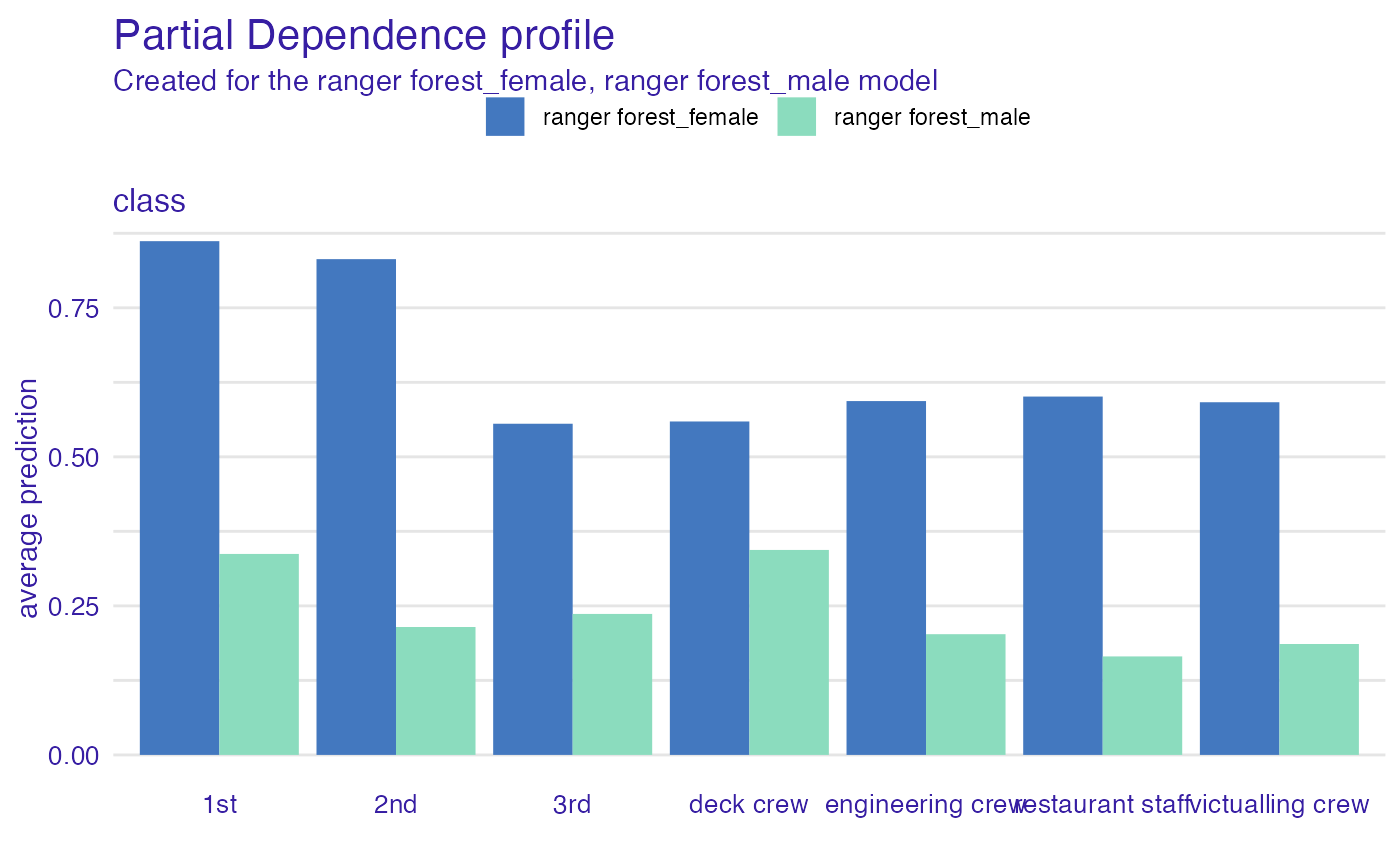# or maybe flipped?
plot(pdp_rf, variables = "class") + coord_flip()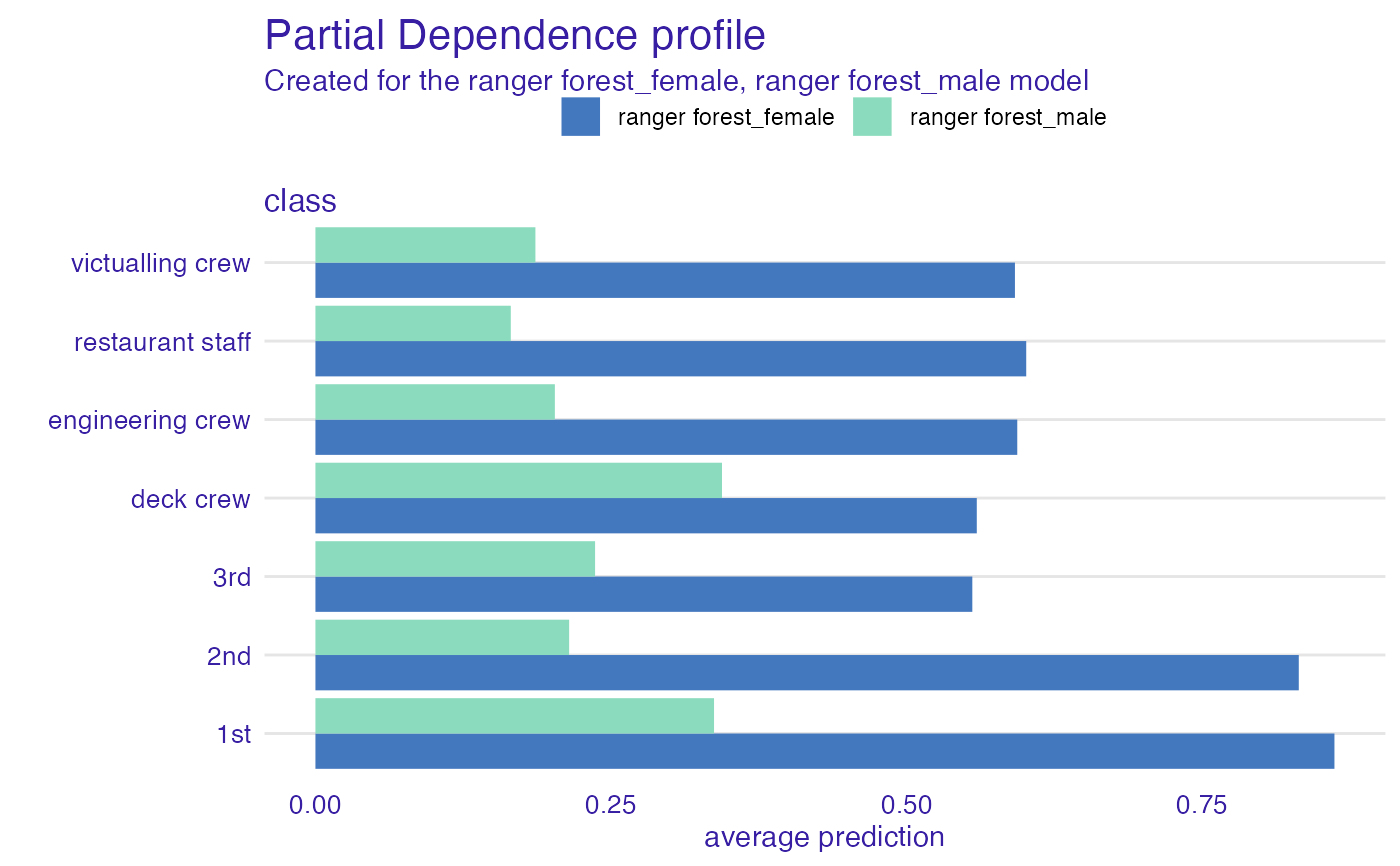# }